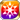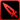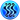# X Marks the SpotDeciding to follow her for her fire and her spirit was my decision, but still... To think that I looked away for but a moment and she's already all the way up there! "This treasure is now the property of the captain of the Xiao Shenmei!" she declares, which tells me that she is, at least, unscathed. She might be my captain, but her terrible sense of direction—or perhaps more accurately, utter lack of any directional sense—continues to flummox me. Well, I can't just leave her up there; if she gets lost, we'll never find her again. I suppose I had better have her hold hands with Su Yi to ensure that she makes it safely back on board the ship. (From Liu Shen's "Treasure Acquisition Log")

## Stats

#### Simple stats

TypeLvl 1Lvl 30Lvl 40
HP+30+67+80

LevelHP
1+30
2+31
3+32
4+33
5+35
6+36
7+37
8+38
9+40
10+41
LevelHP
11+42
12+44
13+45
14+46
15+47
16+49
17+50
18+51
19+53
20+54
LevelHP
21+55
22+56
23+58
24+59
25+60
26+62
27+63
28+64
29+65
30+67
LevelHP
31+68
32+69
33+71
34+72
35+73
36+74
37+76
38+77
39+78
40+80

## Group skills

### Greed Dike's Vitality

#### Simple stats

TypeLvl 1Lvl 30Lvl 40
HP+130+330+400

LevelHP
1+130
2+136
3+143
4+150
5+157
6+164
7+171
8+178
9+185
10+192
LevelHP
11+199
12+206
13+213
14+220
15+226
16+233
17+240
18+247
19+254
20+261
LevelHP
21+268
22+275
23+282
24+289
25+296
26+303
27+310
28+316
29+323
30+330
LevelHP
31+337
32+344
33+351
34+358
35+365
36+372
37+379
38+386
39+393
40+400

#### Limit Break

TypeLB1LB2LB3LB4LB5
HP+48+96+144+192+240

### Greed Dike's Vitality

#### Simple stats

TypeLvl 1Lvl 30Lvl 40
HP+130+330+400

LevelHP
1+130
2+136
3+143
4+150
5+157
6+164
7+171
8+178
9+185
10+192
LevelHP
11+199
12+206
13+213
14+220
15+226
16+233
17+240
18+247
19+254
20+261
LevelHP
21+268
22+275
23+282
24+289
25+296
26+303
27+310
28+316
29+323
30+330
LevelHP
31+337
32+344
33+351
34+358
35+365
36+372
37+379
38+386
39+393
40+400

#### Limit Break

TypeLB1LB2LB3LB4LB5
HP+48+96+144+192+240

### Yo-Ho-Ho

#### Simple stats

TypeLvl 1Lvl 30Lvl 40
Non-type ATK Power+3+8+10
Hit Rate+3+8+10

#### Detailed stats

LevelNon-type ATK PowerHit Rate
1+3+3
2+3+3
3+3+3
4+3+3
5+3+3
6+3+3
7+4+4
8+4+4
9+4+4
10+4+4
LevelNon-type ATK PowerHit Rate
11+4+4
12+4+4
13+5+5
14+5+5
15+5+5
16+5+5
17+5+5
18+6+6
19+6+6
20+6+6
LevelNon-type ATK PowerHit Rate
21+6+6
22+6+6
23+6+6
24+7+7
25+7+7
26+7+7
27+7+7
28+7+7
29+8+8
30+8+8
LevelNon-type ATK PowerHit Rate
31+8+8
32+8+8
33+8+8
34+8+8
35+9+9
36+9+9
37+9+9
38+9+9
39+9+9
40+10+10

#### Limit Break

TypeLB1LB2LB3LB4LB5
Non-type ATK Power+1+2+3+4+6
Hit Rate+1+2+3+4+6

#### Simple stats

TypeLvl 1Lvl 30Lvl 40
Water+3+6+8
Crit Rate+5+12+15
Magic Res+3+6+8

#### Detailed stats

LevelWaterCrit RateMagic Res
1+3+5+3
2+3+5+3
3+3+5+3
4+3+5+3
5+3+6+3
6+3+6+3
7+3+6+3
8+3+6+3
9+4+7+4
10+4+7+4
LevelWaterCrit RateMagic Res
11+4+7+4
12+4+7+4
13+4+8+4
14+4+8+4
15+4+8+4
16+4+8+4
17+5+9+5
18+5+9+5
19+5+9+5
20+5+9+5
LevelWaterCrit RateMagic Res
21+5+10+5
22+5+10+5
23+5+10+5
24+5+10+5
25+6+11+6
26+6+11+6
27+6+11+6
28+6+11+6
29+6+12+6
30+6+12+6
LevelWaterCrit RateMagic Res
31+6+12+6
32+6+12+6
33+7+13+7
34+7+13+7
35+7+13+7
36+7+13+7
37+7+14+7
38+7+14+7
39+7+14+7
40+8+15+8

#### Limit Break

TypeLB1LB2LB3LB4LB5
Water+1+2+3+4+5
Crit Rate+2+3+5+7+9
Magic Res+1+2+3+4+5

TypeLB5
Crit DMG Rate+20

## Vision abilities

### Limit Reached Bonus

#### Ability base

• Slot:Support

Revealed after Limit Reached. Raises AGI after appearing on map.

#### Ability max

• Slot:Action

Raises AGI after appearing on map.

### Chart Wave

#### Ability base

• Slot:Action
• DMG Type:Physical
• Element:Water

Water non-type ATK on enemy unit (Strong vs Fire/Aquatic) & raises Power for Water units from Greed Dike [Range: 4, Height Range: 3]

Skill Effect
TypeMinMax
Attack90%116%
• Charges: 1
• Jewel Cost: 60
• Height: 3
• Select Range: Diamond
• Range: 0-4

#### Ability max

• Slot:Action
• DMG Type:Physical
• Element:Water

Water non-type attack on enemy unit (Strong vs Fire/Aquatic) & raises Power for Water units from Greed Dike [Range: 4, Height Range: 3]

Skill Effect
TypeMinMax
Attack140%140%
• Charges: 1
• Jewel Cost: 60
• Height: 3
• Select Range: Diamond
• Range: 0-4